# Refocusing Amdahl’s Law

Refocusing Amdahl’s Law

Parallelising code can make it faster. Lucian Radu Teodorescu explores how to get the most out of multi-threaded code.

Throwing 10 cores at a serial algorithm doesn’t make it 10 times faster – that’s a well-known fact. Oftentimes, the algorithm barely becomes 3 times faster. Such a waste of resources! And who is to blame? Of course, it must be Gene Amdahl, with his stupid law [ Amdahl67 ] – after all, we spent several months fine-tuning our algorithm to perfection, so we are not to blame.

Now seriously, Amdahl’s law seems to be a universal law that prevents us taking advantage of increasing parallelism in our hardware. It’s similar to how the speed of light constant is limiting progress in microprocessor design.

But, similar to how the hardware industry learned to avoid the restrictions imposed by physics by focusing on other aspects (like multithreading, multicores, caching, etc), the software industry can improve parallelism by changing the focus.

This article explores how we can change our focus in concurrent applications to drastically reduce the negative effects of Amdahl’s law. It mainly aims at moving the focus off lock-based programming.

## Amdahl’s law and related formulas

Amdahl’s law [ Amdahl67 ] gives an upper bound to the maximum amount of speedup one algorithm 1 can obtain by increasing the parallelism level: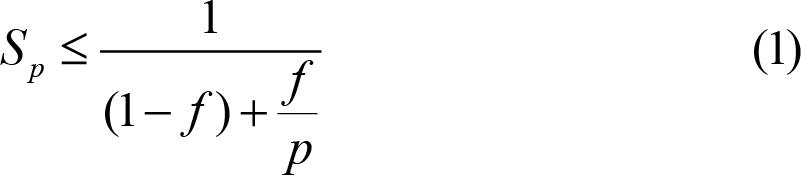where f is the fraction of the code that is parallelizable and P is the parallelism level (number of cores). The formula assumes that the parallelizable part is completely parallelizable.

Figure 1 shows the maximum speedup of an algorithm for parallelism factors of 50%, 80%, 90%, 95%, 99%.Figure 1

A parallelism factor of 90% – that is, 90% of the algorithm can be parallelizable – sounds good at first glance. However, if we plug this value into Amdahl’s formula, we obtain a maximum speedup of 5.26 for 10 cores – roughly half of what we naively expect. If the parallelism factor is 80%, then the speedup for 10 cores would be 3.57 – this doesn’t seem good at all.

If we had an infinite amount of cores, a 90% parallelizable code would have a maximum speedup of 10. Similarly, an 80% parallelizable code would have a maximum speedup of 5. The intuition behind this is simple: no matter how many cores we throw at the problem we can’t improve the serial part; for the 90% parallelizable factor we can’t improve 10% of the code no matter what.

If W ser is the time needed to perform the work that cannot be parallelised at all, and W par is the time needed for the parallelizable work, then the time taken if we have P cores is bounded by T P W ser + W par / P . If P → ∞, then T P W ser .

The time needed in the absence of parallelism, T 1 , is called work . The time needed to run the algorithm if we assume infinite parallelism, T , is called span . Similar to Amdahl’s law, we can use work and span to calculate an upper bound to the speedup, somehow simpler to compute: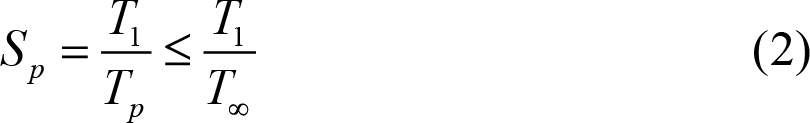In Amdahl’s law, W ser is work that cannot be parallelised at all, while W par is work that can be parallelised to a certain degree. Thus, we obtain an upper bound for speedup. Brent [ Brent74 ] uses a slightly different division to arrive at a lower bound formula. He assumes that T is work that cannot be perfectly parallelizable and, thus, T 1 - T is perfect parallelizable work. With this division he arrives at the following inequality: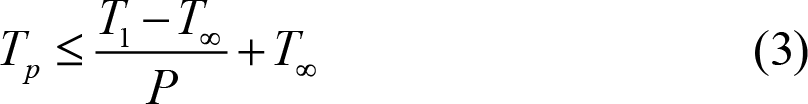This puts a lower bound to the speedup we might have. All these formulas can be used to estimate the parallelism level of an algorithm. See [ McCool12 ] for more theoretical details.

Let us take the example from Figure 2. It represents the work that an algorithm needs to make, with the explicit dependencies between different parts. The yellow (light) work units are on the critical path – they are the span of the algorithm. Assuming all the work units take 1 second to complete, T = 6, while T 1 = 18.Figure 2

According to Amdahl’s view, T 1 and T 18 are not parallelizable, so it overestimates the possible speedup. The inequality with work and span gives an even tighter bound to speedup and Brent’s lemma gives a lower bound to/for the speedup. They are all shown in Figure 3.Figure 3

Let me be very clear on this one: in a multithreaded world, mutexes provide exclusive access to evil .

By design, mutexes block threads from executing certain regions of code; by design, they limit the throughput of the application; by design, they are bottlenecks. The reader should excuse me for putting it as bluntly as I can: after so much has been written on this topic, and after so many talks on this topic (see for example [ Henney18 ], [ Henney17 ], [ Parent17 ], [ Lee06 ]), if someone still believes that mutexes are not inefficiencies/bottlenecks then that person must be very confused. And the same goes with other lock-based primitives.

But the unfortunate reality is that using locks is still mainstream. And these probably have the biggest negative impact on Amdahl’s law.

Whenever we distinguish between parallelizable and non-parallelizable code in an algorithm, we must accept that the non-parallelizable code comes from two main sources:

• Inherently serial code; i.e., sometimes we need to wait for the result of a computation before we can start another computation
• Code serialised through locks.

Of course, the ratio between the two depends on the application, but, in practice, we often find that locks are the main source of non-parallelizable code. In my experience working on an application that should have a sub-second reaction time, I’ve encountered (multiple times) cases in which threads are waiting to acquire locks for more than two minutes, and also cases in which lock chains have involved more than 10 locks (a lock waits on a lock, which waits on another lock, and so on). Typically, as soon as people add locks to a project, the performance quickly goes south.

We add locks to make our code thread-safe . This thread-safe terminology gives us a sense that everything is ok, including performance – and, of course, it’s not. Instead, we should be saying that we add locks to become thread-adverse .

Let’s assume that we have a unit of work that takes 1 second to execute. When there is no contention, the impact of adding the locks is minimal – and probably most people just measure this scenario. But, in the presence of contention, that unit of work can take 10%, 50%, or even 100% more time to execute. In Figure 4, we have an example of running a unit of work with locks in parallel; the example shows a performance degradation of 20% and 30%. And, what is worse, we may not be hitting all the non-parallelisable code in the example. Thus the factor in Amdahl’s law can be even less than 70%. This can be a big impediment to scaling.Figure 4

Another problem that we often encounter in the classical multithreaded world is the static allocation of work per thread. Applications, from build time, are configured with a fixed number of threads. Of course, if the machine has more cores than the number of threads, the application cannot properly scale. But also the opposite case can hurt performance: having too many threads on a limited number of cores can make the performance worse (i.e., because of thread switching and cache effects). Besides these two extremes, the static allocation of work may cause periods of times with not enough work.

So, in the classical multithreaded world, it’s very easy to reach cases in which the ratio of parallelizable code is reduced, thus affecting the speedup of the application.

## A change of perspective

To obtain significant speedups, we need to combat the negative effects of Amdahl’s law (which provides an upper bound) and to take more advantage on Brent’s lemma (which guarantees us a lower bound). That is, we need to reduce the amount of serial code, and increase the work/span ratio.

We would want to ensure that there is no contention between two units of work (tasks) that run in parallel, and moreover, at any given point in time, we have enough (better: the right amount) such tasks to execute. This is achievable by following a relatively simple set of rules:

• we break the algorithm into a set of tasks; a task is an independent unit of work
• we add constraints between tasks, modelling dependencies and possible race conditions
• we use a dynamic execution strategy for tasks; one worker thread per core
• we ensure that the algorithm is decomposed in enough tasks at every given point in time

The key here is the definition of tasks: the unit of work is independent . That is, two active tasks should never block each other. If two tasks are conflicting, they cannot be active at the same time. That is why we need to add constraints between tasks (second bullet). And, as the constraints between the tasks would have to be dynamically set (as we want our worker threads to always work on the next available task), we need to have a dynamic execution of our tasks. And, a simple greedy execution would work well in practice, as it would maximise the amount of work being executed.

And with that, we roughly solved the contention part between tasks. At this point I would ask the reader to accept that it is feasible, in practice, to implement such a system; I will not try to prove that we can always restructure the algorithms this way – let’s leave this to a follow-up article.

The other part, ensuring that the work/span ratio is large enough, can be done through proper decomposition of the problem. Unfortunately, there is no general algorithm to solve this. There are a series of patterns that can be applied to parallel programming (a good catalogue of patterns can be found in [ McCool12 ]), and experience so far tells us that this decomposition is doable in practice for most problems.

If both of these conditions are met, and for most of the problems can be met, we can count on a significant speedup. But let’s work out the details.

## Deriving the new formula

First, let us consider a simplified case in which, for the whole duration of the algorithm, we have running tasks, and we run them continually. This is depicted in Figure 5. We code with yellow the actual duration of the task and with red the time spent in the framework that executes tasks (overhead compared to static allocation of work).Figure 5

The tasks are, by design, fully parallelizable, but in the real world they always have some indirect contention (i.e., caching effects, memory allocation, OS calls, etc.). But, let’s assume for now that they can achieve perfect parallelism. That is, in our figure, at any point in which we have yellow bars we are achieving perfect parallelisation. After executing each task, we need to add some logic in the execution framework to choose the next task to execute and actually start executing it. Depending on the implementation of the framework code, this can be more or less parallelizable (a good implementation would have very low contention for average use cases). Let us denote this time as W fw = αW tasks , assuming that it’s a fraction of the time spent executing tasks.

If f 1 is the parallelisation ratio of the work associated with the tasks (considering the indirect contention), and f 2 is the parallelisation ratio for the task framework code, then the fully parallelizable part would be W par = W tasks ( f 1 + α f 2 ) / (1 + α), while the non-parallelizable part would be W ser = W tasks (1 - ( f 1 + α f 2 ) / (1 + α)). Plugging this into Amdahl’s law, we obtain: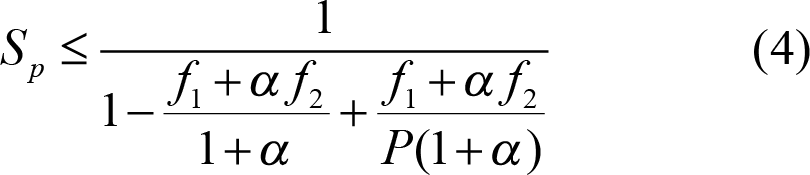To be able to make sense of this formula, let’s consider for the moment that f 1 = 1. Yes, this is not true in practice – but for this discussion, it is a good convention. We can consider that this is an inherent limitation in software construction, one that largely cannot be avoided. So, to assess the limits of our task-based system, we should not consider factors that are not controllable. It’s similar to ignoring speed of light for non-relativistic mechanics.

Moving forward, to give an example, we can consider f 2 = 0.5, and α to be 1⁄1000 (i.e., on my machine I have a task system that has an overhead of the order of microseconds, per task). That would give us a general factor in Amdahl’s law of 0.9995005. That is, for 1000 cores we would obtain a maximum speedup of 667. For 10 cores, the speedup limit would be 9.955. That is a great result! (deliberately ignoring indirect parallelism)

Ok, now it’s time to consider the second limiting factor: we don’t always have tasks to run. When we start the algorithm, we typically have only one task running; that task then creates and spawns the tasks that can be executed in parallel. Also, there are problems that may not expose high degrees of parallelism, so at various points in time, the number of available tasks can be less than the number of cores (undersubscription).

We would introduce β to be the ratio between the time we have more tasks than cores and the total time of the algorithm. With this, the global Amdahl’s factor becomes: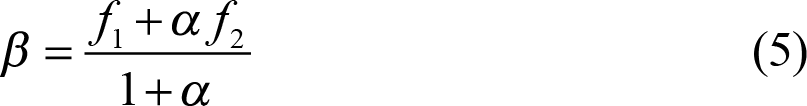and the new formula: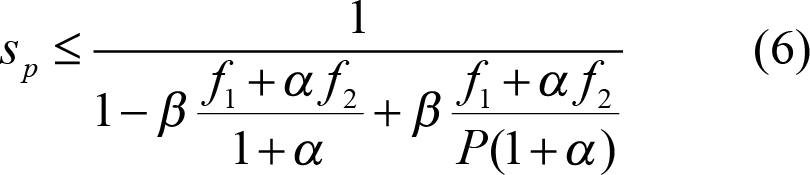At this point, we can also argue that, for certain algorithms and applications, we can make β be practically 1. If we care about the total application throughput, then the fact that our algorithm does have enough tasks at a given point may be irrelevant if there are other parts of the application with tasks. So, if we succeed in scheduling enough tasks around the algorithm, we can practically consider that β = 1.

Another argument for discounting the effect of the β factor relates to an assumption in Amdahl’s law: we always assume that the total amount of work is constant, thus we measure against a constant work. But, whenever we discuss scalability and throughput, we oftentimes want to increase the problem size. That is, we are not interested in how much faster we can make one single computation of 1 second in complete isolation. We often consider the throughput of making many such computations. This means that we could have enough tasks at any time to fill the available cores.

That is, if we measure the speedup of the algorithm inside an application that is designed to maximise throughput, we can theoretically obtain a speedup of 667 for 1000 cores and 9.995 for 10 cores.

This is the upper limit as given by Amdahl’s law. If we can shoot for that it’s very good… let’s shoot for that. But to do so, let us turn our attention to the lower limit of speedup given by Brent’s lemma.

To simplify our calculation, let’s assume that our work is divided in N equal tasks: W tasks = N W 0 . The amount of task framework work that needs to be done is also proportional to N , so the total work is T 1 = N ( W 0 + Wfw 0 ). If we need K tasks for our span, then T = K ( W 0 + Wfw 0 ). This means that T 1 = N / K T , and thus T P ≤ ( W 0 + Wfw 0 )(1+( N / K - 1)/ P ). This would give us a speedup of: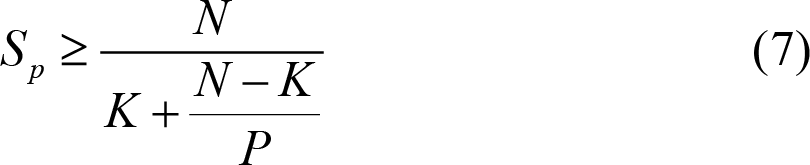If we are targeting high-throughput, and we can hide the latency of the computation among a multitude of other computations, we can practically consider K = 1, and thus the formula becomes:Again, this formula ignores some the fact that, in practice, it is hard to find perfect parallelisation, so it must be used with care.

This formula is important, as it gives us a guaranteed speedup (under all the assumptions we considered). For example, if N =1000, we have a minimum speedup of 500.25 for 1000 cores, and a minimum speedup of 9.91 for 10 cores).

I believe that these numbers will make most readers want to drop lock-based multithreaded programming and embrace task-based programming. If that’s the case, I am personally very happy.

But before we directly jump on task-based programming, a contextualisation is needed.

## Discussion

Having enough tasks to run. A key assumption we’ve made when deriving the above formula is that we have enough tasks to run at any given time; to be more precise, more than the number of available cores. This is known in the literature as overdecomposition [ McCool12 ] – decomposing the problem into more tasks than we need. In multithreaded contexts, we should always aim for overdecomposition, but this is not always possible.

A major problem is that, even though that algorithm might have enough tasks overall, there may be times in which the algorithm doesn’t have enough tasks to execute. This is mainly induced by the constraints we need to have on our tasks. If, for example, an algorithm has a long task that would spawn other tasks just at the end, then the algorithm would have times with undersubscription, which would hurt scalability.

Too much decomposition. From the point of view of this article, overdecomposition is highly encouraged. However, in practice, there are also costs associated with it. One cannot generate a near-infinite number of tasks. Tasks may be bound to resources (i.e., they need memory or special initialisation code) and we cannot afford to create too many of these tasks. This heavily depends on the algorithm being solved. For example, for an h264 video decoding a task, decoding a frame might need a context to run into; creating such a context might not be very cheap. So, applications may also want to limit the amount of decomposition.

High-throughput scenarios. The whole article focuses on maximising throughput. But, as we well know, maximising throughput can lead to increased latency of the application. So, the advice of this article may not apply to low-latency applications.

Fixed work vs scalable work. Most of the formulas in this article revolve around the total work that an algorithm needs to do, but they are not very explicit. For example, Amdahl’s law assumes a fixed amount of work; running the algorithm on more cores should not change the amount of work done. But this is generally not true in practice. First, moving from a single-threaded application to a multi-threaded application implies more work. Secondly, the amount of work is typically determined at runtime based on the actual execution trace. For the sake of simplicity, I left this discussion outside the article.

Beware of indirect contention. If we remove locks from our multithreading programming, indirect contention might become visible. And the more parallelism we add to our programs, the more this will be a problem. There are two parts on indirect contention: one inside our software (I/O, memory allocator, OS calls, use of atomics 2 etc.), and one outside of our software (operating system, hardware, other equipment, etc.). For example, the hyperthreading feature on a processor can limit the processing power as two threads can be fighting on the resources of the same core.

## Conclusions and next steps

No matter what we do, we can’t completely eliminate the limitations imposed by Amdahl’s law; there are always factors that would limit the speedup of our code. But, we can shift our focus. Instead of focusing on external limitations in our software, we can change the paradigm in which we are writing multithreaded software. Changing this focus, we can eliminate the heavy intrinsic limitations of Amdahl’s law.

If we focus on eliminating the locks from our software and we replace them with tasks, then, provided that we have enough tasks to be run at any given time and that the overhead of the task execution framework is small, we can achieve very good speedups. We analyse the upper bound speedup (starting from Amdahl’s law) and the lower bound of the speedup (starting from Brent’s lemma). In both cases, we converge to speedups that are bigger to what we typically see in current practice.

The one big question that the article doesn’t explore is whether we can move to tasks for any type of problems. It only previews the main principles that would make the system work, but doesn’t provide solid arguments on how this can be done. I can only assume that the reader is not fully convinced that such a system would be feasible for most applications. And that just binds me to write another article on this topic.

Until that time, the reader should at least start to be suspicious of locks found in the code. As a good tip, follow Kevlin Henney’s advice and call all the mutexes ‘bottlenecks’ – after all it’s the truth.

## Appendix. A small example

I cannot finish this article without giving a code sample, without putting the theory into practice. Let us use a task-based system to compute the Mandelbrot fractal. The concurrency of the algorithm is implemented with the help of the Concore library [ concore ]. See Listing 1. (I started to write this library to help me understand how to better write concurrent programs).

 ```int mandel_core(std::complex c, int depth) { int count = 0; std::complex z = 0; for (int i = 0; i < depth; i++) { if (abs(z) >= 2.0) break; z = z * z + c; count++; } return count; } void serial_mandel(int* vals, int max_x, int max_y, int depth) { for (int y = 0; y < max_y; y++) { for (int x = 0; x < max_x; x++) { vals[y * max_x + x] = mandel_core(transform(x, y), depth); } } } void parallel_mandel(int* vals, int max_x, int max_y, int depth, concore::task& done) { std::atomic remain{max_y}; for (int y = 0; y < max_y; y++) { concore::global_executor([=, &remain, &done](){ for (int x = 0; x < max_x; x++) { vals[y * max_x + x] = mandel_core(transform(x, y), depth); } if (remain-- == 1) concore::global_executor(done); }); } } ``` Listing 1

The problem is relatively simple: we have a matrix that needs to be filled with the number of steps until the series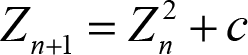diverges (well, approximated) [ Wikipedia ]. The elements are completely independent of each other. That allows us a straight-forward parallelisation. We chose to (explicitly) create a task for each row of the matrix. The reader will pardon my use of atomic here; it’s a source of indirect contention, but I just wanted to keep this simple.

Running a simple benchmark on my MacBook Pro 16″ 2019, 2.6 GHz 6-Core Intel Core i7, I get the results shown in Figure 6. Considering all the disclaimers we’ve made during this article (not a perfect throughput test, indirect contention on memory, indirect contention with other processes, hardware, etc.) the results are in line with our calculations for up to 6 cores – which is also the number of non-hyperthreading cores I have on my machine (this is a proof that hyperthreading is a big source of indirect contention).Figure 6

Speaking of indirect contention that cannot be controlled from software: during the run of the tests the operating frequency of my CPU drops as the number of worker threads go up. We just hit the limitations outside of software. And that just shows that focusing on obtaining the perfect Amdahl formula is a losing strategy.

## References

[Amdahl67] Gene M. Amdahl (1967) Validity of the Single Processor Approach to Achieving Large-Scale Computing Capabilities , AFIPS Conference Proceedings (30): 483–485 (Proceedings of the 18–20 April 1967 joint computer conference.)

[Brent74] R.P. Brent, ‘The parallel evaluation of general arithmetic expressions’, Journal of the Association for Computing Machinery , 21(2), 1974.

[concore] Lucian Radu Teodorescu, Concore library: https://github.com/lucteo/concore

[Henney17] Kevlin Henney, ‘Thinking Outside the Synchronisation Quadrant’, ACCU 2017 , available at: https://www.youtube.com/watch?v=UJrmee7o68A

[Henney18] Kevlin Henney, ‘Concurrency Versus Locking’ 2 Minute Tech Tip , available at: https://www.youtube.com/watch?v=mEtoXwB9HFk

[Lee06] Edward A. Lee (2006) The Problem with Threads , Technical Report UCB/EECS-2006-1, available at: https://www2.eecs.berkeley.edu/Pubs/TechRpts/2006/EECS-2006-1.pdf

[McCool12] Michael McCool, Arch D. Robison, James Reinders, Structured Parallel Programming: Patterns for Efficient Computation , Morgan Kaufmann, 2012

[Parent17] Sean Parent, Better Code: Concurrency , NDC London 2017, available at: https://www.youtube.com/watch?v=zULU6Hhp42w

[Wikipedia] Mandelbrot set: https://en.wikipedia.org/wiki/Mandelbrot_set

1. I’m using the term algorithm here to denote the work that we consider parallelism for. This may not be the full program/application, but, by convention, we assume it’s a significant part of it. I wanted to distinguish it from application , which contains one or multiple invocations of the algorithm . Beside the invocation of the algorithm , the application may have other parts that are not relevant for improving parallelism (e.g., startup, shutdown). Also, the use of the term algorithm should not be confused with implementations of well-known algorithms (like the ones found in standard libraries); we should use the general form (finite well-defined set of steps).
2. At the moment, I still use atomics in my day-to-day code while heavily avoiding locks. But atomics have essentially the same downsides as the locks do, just at a much finer scale. My hope is that after we are good at removing locks from our code, the next step would be to find systematic ways of removing the need for using atomics in high-level code.

has a PhD in programming languages and is a Software Architect at Garmin. As hobbies, he is working on his own programming language and he is improving his Chuck Norris debugging skills: staring at the code until all the bugs flee in horror.